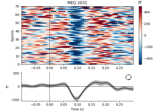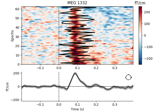# mne.viz.plot_epochs_image#

mne.viz.plot_epochs_image(epochs, picks=None, sigma=0.0, vmin=None, vmax=None, colorbar=True, order=None, show=True, units=None, scalings=None, cmap=None, fig=None, axes=None, overlay_times=None, combine=None, group_by=None, evoked=True, ts_args=None, title=None, clear=False)[source]#

Plot Event Related Potential / Fields image.

Parameters:
epochsinstance of `Epochs`

The epochs.

picks

Channels to include. Slices and lists of integers will be interpreted as channel indices. In lists, channel type strings (e.g., ```['meg', 'eeg']```) will pick channels of those types, channel name strings (e.g., `['MEG0111', 'MEG2623']` will pick the given channels. Can also be the string values “all” to pick all channels, or “data” to pick data channels. None (default) will pick good data channels. Note that channels in `info['bads']` will be included if their names or indices are explicitly provided. `picks` interacts with `group_by` and `combine` to determine the number of figures generated; see Notes.

sigma`float`

The standard deviation of a Gaussian smoothing window applied along the epochs axis of the image. If 0, no smoothing is applied. Defaults to 0.

vmin

The min value in the image (and the ER[P/F]). The unit is µV for EEG channels, fT for magnetometers and fT/cm for gradiometers. If vmin is None and multiple plots are returned, the limit is equalized within channel types. Hint: to specify the lower limit of the data, use `vmin=lambda data: data.min()`.

vmax

The max value in the image (and the ER[P/F]). The unit is µV for EEG channels, fT for magnetometers and fT/cm for gradiometers. If vmin is None and multiple plots are returned, the limit is equalized within channel types.

colorbarbool

Display or not a colorbar.

order

If not `None`, order is used to reorder the epochs along the y-axis of the image. If it is an array of `int`, its length should match the number of good epochs. If it is a callable it should accept two positional parameters (`times` and `data`, where `data.shape == (len(good_epochs), len(times))`) and return an `array` of indices that will sort `data` along its first axis.

showbool

Show figure if True.

units

The units of the channel types used for axes labels. If None, defaults to `units=dict(eeg='µV', grad='fT/cm', mag='fT')`.

scalings

The scalings of the channel types to be applied for plotting. If None, defaults to ```scalings=dict(eeg=1e6, grad=1e13, mag=1e15, eog=1e6)```.

cmap`None` | colormap | (colormap, bool) | ‘interactive’

Colormap. If tuple, the first value indicates the colormap to use and the second value is a boolean defining interactivity. In interactive mode the colors are adjustable by clicking and dragging the colorbar with left and right mouse button. Left mouse button moves the scale up and down and right mouse button adjusts the range. Hitting space bar resets the scale. Up and down arrows can be used to change the colormap. If ‘interactive’, translates to (‘RdBu_r’, True). If None, “RdBu_r” is used, unless the data is all positive, in which case “Reds” is used.

fig

`Figure` instance to draw the image to. Figure must contain the correct number of axes for drawing the epochs image, the evoked response, and a colorbar (depending on values of `evoked` and `colorbar`). If `None` a new figure is created. Defaults to `None`.

axes

List of `Axes` objects in which to draw the image, evoked response, and colorbar (in that order). Length of list must be 1, 2, or 3 (depending on values of `colorbar` and `evoked` parameters). If a `dict`, each entry must be a list of Axes objects with the same constraints as above. If both `axes` and `group_by` are dicts, their keys must match. Providing non-`None` values for both `fig` and `axes` results in an error. Defaults to `None`.

overlay_timesarray_like, shape (n_epochs,) | `None`

Times (in seconds) at which to draw a line on the corresponding row of the image (e.g., a reaction time associated with each epoch). Note that `overlay_times` should be ordered to correspond with the `Epochs` object (i.e., `overlay_times` corresponds to `epochs`, etc).

combine

How to combine information across channels. If a `str`, must be one of ‘mean’, ‘median’, ‘std’ (standard deviation) or ‘gfp’ (global field power). If callable, the callable must accept one positional input (data of shape `(n_epochs, n_channels, n_times)`) and return an `array` of shape `(n_epochs, n_times)`. For example:

```combine = lambda data: np.median(data, axis=1)
```

If `combine` is `None`, channels are combined by computing GFP, unless `group_by` is also `None` and `picks` is a list of specific channels (not channel types), in which case no combining is performed and each channel gets its own figure. See Notes for further details. Defaults to `None`.

group_by

Specifies which channels are aggregated into a single figure, with aggregation method determined by the `combine` parameter. If not `None`, one `Figure` is made per dict entry; the dict key will be used as the figure title and the dict values must be lists of picks (either channel names or integer indices of `epochs.ch_names`). For example:

```group_by=dict(Left_ROI=[1, 2, 3, 4], Right_ROI=[5, 6, 7, 8])
```

Note that within a dict entry all channels must have the same type. `group_by` interacts with `picks` and `combine` to determine the number of figures generated; see Notes. Defaults to `None`.

evokedbool

Draw the ER[P/F] below the image or not.

ts_args

Arguments passed to a call to `plot_compare_evokeds` to style the evoked plot below the image. Defaults to an empty dictionary, meaning `plot_compare_evokeds` will be called with default parameters.

title

If `str`, will be plotted as figure title. Otherwise, the title will indicate channel(s) or channel type being plotted. Defaults to `None`.

clearbool

Whether to clear the axes before plotting (if `fig` or `axes` are provided). Defaults to `False`.

Returns:
figs

One figure per channel, channel type, or group, depending on values of `picks`, `group_by`, and `combine`. See Notes.

Notes

You can control how channels are aggregated into one figure or plotted in separate figures through a combination of the `picks`, `group_by`, and `combine` parameters. If `group_by` is a `dict`, the result is one `Figure` per dictionary key (for any valid values of `picks` and `combine`). If `group_by` is `None`, the number and content of the figures generated depends on the values of `picks` and `combine`, as summarized in this table:

group_by

picks

combine

result

dict

None, int, list of int, ch_name, list of ch_names, ch_type, list of ch_types

None, string, or callable

1 figure per dict key

None

None, ch_type, list of ch_types

None, string, or callable

1 figure per ch_type

int, ch_name, list of int, list of ch_names

None

1 figure per pick

string or callable

1 figure

## Examples using `mne.viz.plot_epochs_image`#XDAWN Denoising

XDAWN DenoisingVisualize channel over epochs as an image

Visualize channel over epochs as an image